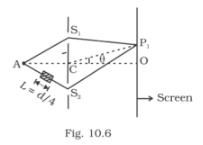Deepak Scored 45->99%ile with Bounce Back Crack Course. You can do it too!

# AC = CO = D, S1 C = S2 C = d << D A small transparent slab containing material

Question:

AC = CO = D, S1 C = S2 C = d << D A small transparent slab containing material of µ =1.5 is placed along AS2. What will be the distance from O of the principal maxima and of the first minima on either side of the principal maxima obtained in the absence of the glass slab?Solution:

∆x = 2d sin θ+(μ-1)L

sin θ0 = -1/16

From central maxima, OP = -D/16

$\sin \theta_{1}=\frac{\pm \lambda / 2-d / 8}{2 d}$

On the positive side,

$\sin \theta_{1}^{+}=3 / 16$

On the negative side,

$\sin \theta_{1}^{-}=-5 / 16$

The first principal maxima on the positive side is at a distance, which is above point O

$D \tan \theta_{1}^{-}=\frac{3 D}{\sqrt{247}}$

The first principal minima on the negative side is at distance, which is below point O

$D \tan \theta_{1}^{-}=\frac{5 D}{\sqrt{16^{2}-5^{2}}}=\frac{5 D}{\sqrt{231}}$